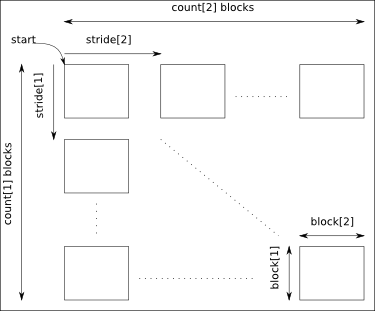Scilab Home page | Wiki | Bug tracker | Forge | Mailing list archives | ATOMS | File exchange
Change language to: English - Português - 日本語 - Русский
Aide de Scilab >> HDF5 Management > h5write

# h5write

Create a dataset (if it does not exist) and write the data

### Syntax

```h5write(obj, name, data [, targetType], [, start, count [, stride [, block]]])
h5write(filename, name, data [, targetType], [, start, count [, stride [, block]]])```

### Arguments

obj

a H5Object

name

a string giving the path to the dataset

data

a Scilab data

targetType

a string giving the type of the target

start

a row of doubles containing the start of the hyperslab

count

a row of doubles containing the count of the hyperslab

stride

a row of doubles containing the stride of the hyperslab

block

a row of doubles containing the block of the hyperslab

filename

a string giving the filename

### Description

Create a new named dataset (if it does not exist) based on the Scilab data passed as argument.

The target HDF5 type can be chosen in the list available in the HDF5 manual. Examples of this HDF5 type are "H5T_MIPS_U32" or "H5T_STD_B64BE", but shortcuts as "MIPS_U32" or "STD_B64BE" can also be used.

It is possible to make an hyperslab selection on the data.The arguments start, count, stride and block must have a size equal to the number of dimensions of the data:

• start: gives the coordinates in the data where to start the selection.
• count: gives the number of selected blocks in each dimension.
• stride: gives the shift between two consecutives blocks in each dimension. Take care that the stride must be greater than the corresponding block dimension.
• block: gives the block dimensions.
By default stride and block are set to 1 in each dimension.

If the target type is "H5T_STD_REF_OBJ", the data matrix must be a string matrix containing the absolute paths of group or datasets.

### Examples

```x = matrix(1:20, 4, 5);
save(TMPDIR + "/x.sod", "x"); // SOD files are HDF5 ones

// Open the created file
a = h5open(TMPDIR + "/x.sod");

// We want to add a new dataset named "y"
y = uint32(matrix(10:30, 7, 3));
h5write(a, "y", y);

// We add a dataset "z" in using MIPS representation
h5write(a, "z", y, "H5T_MIPS_U32");

// Now we make an hyperslab selection
x = uint32(matrix(1:(11*17), 11, 17));
h5write(a, "t", x, [1 2], [2 4], [5 3], [3 2]);

//Now check that all is ok
x, a("/t").data'

// We have finished so we free all the resources
h5close(a);```

### History

 Version Description 5.5.0 HDF5 module introduced.